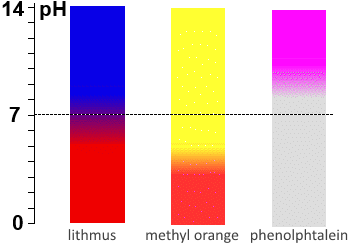Search:

# Acide-base titration

## The equivalence point

### Definition

The equivalence point of a titration (E.p.) is the point where the number of moles $n_1$ of titrant 1 added is just equal to the number of moles $n_2$ of titrated 2 present initially .

### Calculation

Reactive 1 is titrated by reactive 2: On the E.p. we have: $n_1=n_2$ $c_1 \cdot V_1$ $=$ $c_2 \cdot V_2$ $c_1$ $=$ $\frac{c_2 \cdot V_2}{V_1}$ Knowing the molarity of titrant $c_2$ and the volume $V_1$ of the titrated reagent 1, we detect the volume of titrant used on the E.p. to know the molarity of the titrated reagent $c_1$.

At the equivalence point: $c_1$ $=$ $\frac{c_2 \cdot V_2}{V_1}$

### Detection of the equivalent point

The equivalence point is detected: a) by recording the titration curve using a $pH$meter. The $pH$ jump on E.p. is detectable accurately if the base and the acid are strong enough and if the dilutions are no too extensive. b) by conductivity. Before or after E.p. the medium contains hydronium or hydroxide ions in excess. These ions are generally smaller and therefore more mobile than ions present in E.p. and therefore conduct electricity easily. (Transport current in ionic solutions = Migration of ions): The E.p. is characterized by a minimum of the electrical conductivity. c) by color indicators. We require an appropriate indicator so that the turn is made as accurately as possible at the equivalent point.The figure shows the titration curve of a solution of $10\; mL$ $NaOH$ $0.1\; mol/L$ solution by $H Cl$ $0.1\; mol/L$. The turning areas of three colored indicators are shown. We see that - phenolphthalein turns (d1 domain) before we have added the required volume of acid to reach the E.p. $(10 mL)$, - thymol blue turns long after (d2 domain). - the appropriate indicator here is lithmus whose $pKa$ is around 7. Recall that the turning domain of indicators focuses on the value of their $pKa$.Titration of a strong base by a strong acid: The $pH$ of the E.p. remains at 7, so lithmus and bromothymol blue are the best indicators. Titration of a weak acid by a strong base: On the E.p. of a titration of weak acids with strong bases the $pH$ often ranges above 7 because at that time the medium contains only the weak base corresponding to the weak acid of departure: In principle, one should choose the indicator whose $pKa$ is closest to the calculated $pH$ for the E.p., but in practice, phenolphthalein is often indicated. Titration of a weak base by a strong acid: On the E.p. of a titration of weak bases with strong acids the pH often falls below 7 because at that time the medium contains only the weak acid corresponding to the weak base of departure: In principle, one should choose the indicator whose $pKa$ is closest to the calculated $pH$ for the E.p., but in practice, methyl orange is often indicated.